Documentation

### This is machine translation

Mouseover text to see original. Click the button below to return to the English version of the page.

## Control of a Multi-Input Single-Output Plant

This example shows how to design model predictive controller with one measured output, one manipulated variable, one measured disturbance, and one unmeasured disturbance in a typical workflow.

### Define Plant Model

The discrete time linear open-loop dynamic model is defined below with sample time `Ts`.

```sys = ss(tf({1,1,1},{[1 .5 1],[1 1],[.7 .5 1]})); Ts = 0.2; model = c2d(sys,Ts); ```

### Design MPC Controller

Define type of input signals: the first signal is a manipulated variable, the second signal is a measured disturbance, the third one is an unmeasured disturbance.

```model = setmpcsignals(model,'MV',1,'MD',2,'UD',3); ```

Create the controller object with sampling period, prediction and control horizons.

```mpcobj = mpc(model,Ts,10,3); ```
```-->The "Weights.ManipulatedVariables" property of "mpc" object is empty. Assuming default 0.00000. -->The "Weights.ManipulatedVariablesRate" property of "mpc" object is empty. Assuming default 0.10000. -->The "Weights.OutputVariables" property of "mpc" object is empty. Assuming default 1.00000. ```

Define constraints on the manipulated variable.

```mpcobj.MV = struct('Min',0,'Max',1,'RateMin',-10,'RateMax',10); ```

For unmeasured input disturbances, its model is an integrator driven by white noise with variance = 1000.

```mpcobj.Model.Disturbance = tf(sqrt(1000),[1 0]); ```

### Simulate Closed-Loop Response Using the `sim` Command

Specify simulation parameters.

```Tstop = 30; % simulation time Tf = round(Tstop/Ts); % number of simulation steps r = ones(Tf,1); % reference signal v = [zeros(Tf/3,1);ones(2*Tf/3,1)]; % measured disturbance signal ```

Run the closed-loop simulation and plot results.

```sim(mpcobj,Tf,r,v) ```
``` Assuming no disturbance added to measured output channel #1. -->The "Model.Noise" property of the "mpc" object is empty. Assuming white noise on each measured output channel. ```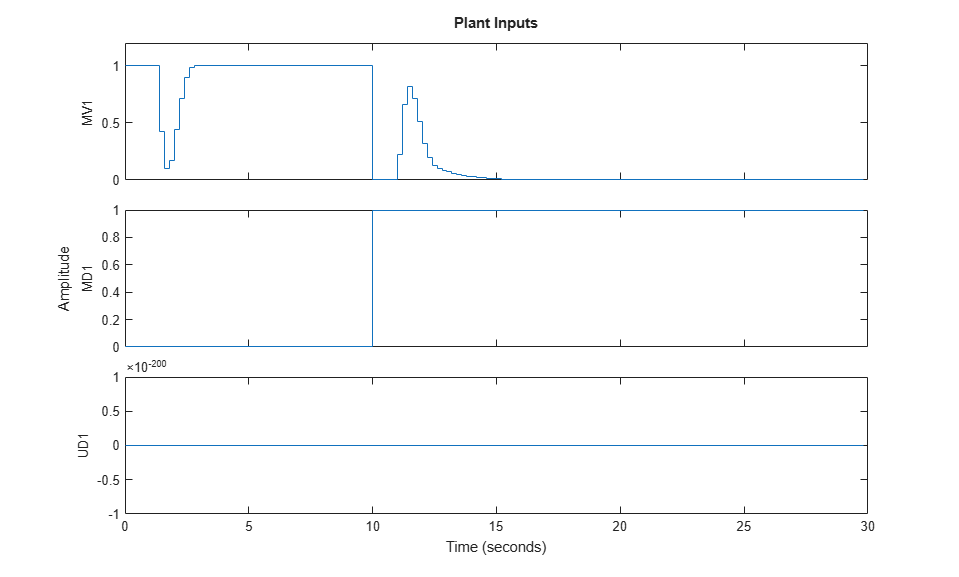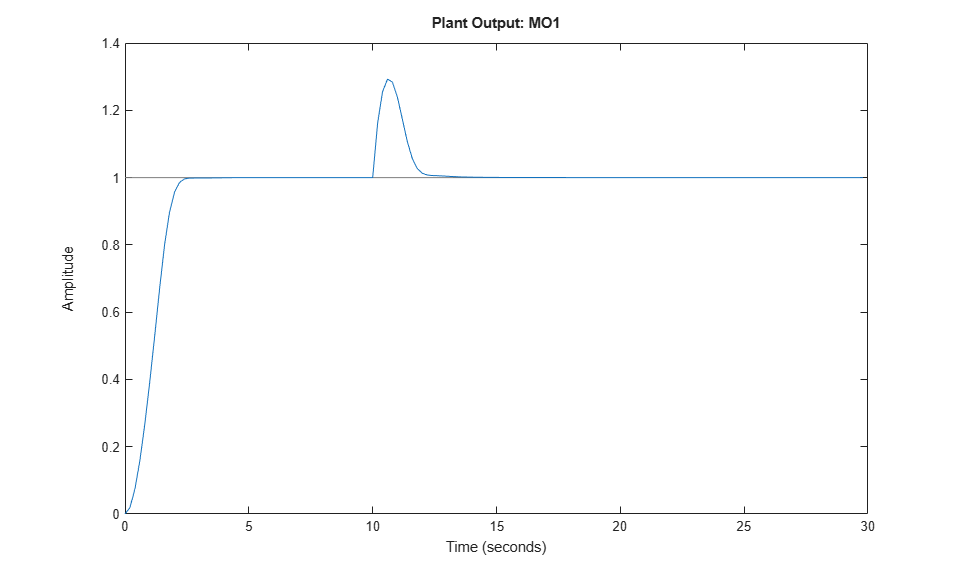Specify disturbance and noise signals in simulation option object.

```SimOptions = mpcsimopt(mpcobj); d = [zeros(2*Tf/3,1);-0.5*ones(Tf/3,1)]; SimOptions.Unmeas = d; % unmeasured input disturbance signal SimOptions.OutputNoise=.001*(rand(Tf,1)-.5); % output measurement noise SimOptions.InputNoise=.05*(rand(Tf,1)-.5); % noise on manipulated variables ```

Run the closed-loop simulation and save the results to the workspace.

```[y,t,u,xp] = sim(mpcobj,Tf,r,v,SimOptions); ```

Plot results.

```figure subplot(2,1,1) plot(0:Tf-1,y,0:Tf-1,r) title('Output') grid subplot(2,1,2) plot(0:Tf-1,u) title('Input') grid ```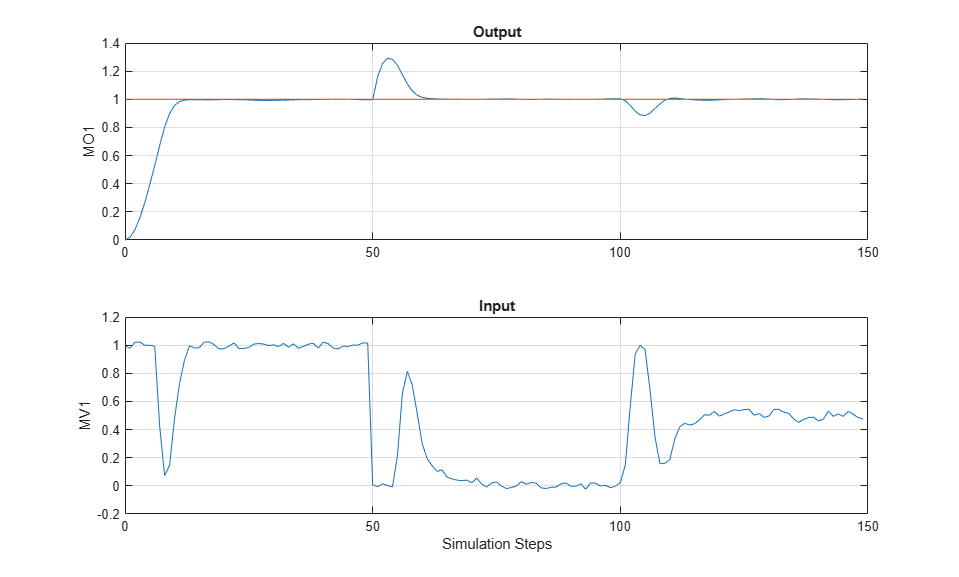### Simulate Closed-Loop Response with Model Mismatch

Test the robustness of the MPC controller against a model mismatch. Specify the true plant `simModel`, with a nominal output value of `0.1`.

```simModel = ss(tf({1,1,1},{[1 .8 1],[1 2],[.6 .6 1]})); simModel = setmpcsignals(simModel,'MV',1,'MD',2,'UD',3); simModel = struct('Plant',simModel); simModel.Nominal.Y = 0.1; simModel.Nominal.X = -.1*[1 1 1 1 1]; ```

Create option object.

```SimOptions.Model = simModel; SimOptions.plantinit = [0.1 0 -0.1 0 .05]; % Initial state of the true plant SimOptions.OutputNoise = []; % remove output measurement noise SimOptions.InputNoise = []; % remove noise on manipulated variables % Run the closed-loop simulation and plot results. sim(mpcobj,Tf,r,v,SimOptions) ```
```-->Converting model to discrete time. ```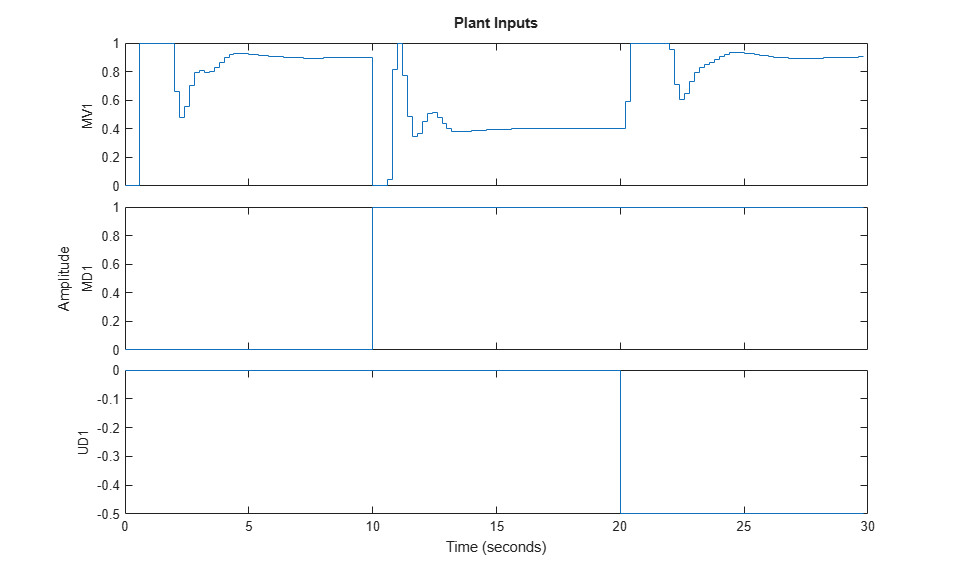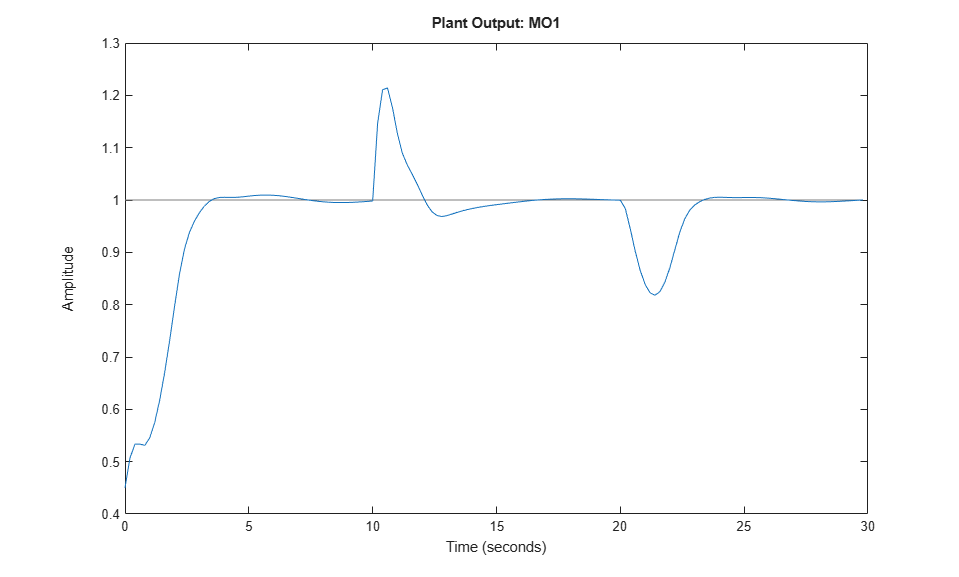### Soften Constraints

Relax the constraints on manipulated variables from hard to soft.

```mpcobj.MV.MinECR = 1; mpcobj.MV.MaxECR = 1; ```

Define an output constraint.

```mpcobj.OV.Max = 1.1; ```

Run a new closed-loop simulation.

```sim(mpcobj,Tf,r,v) ```
``` Assuming no disturbance added to measured output channel #1. -->The "Model.Noise" property of the "mpc" object is empty. Assuming white noise on each measured output channel. ```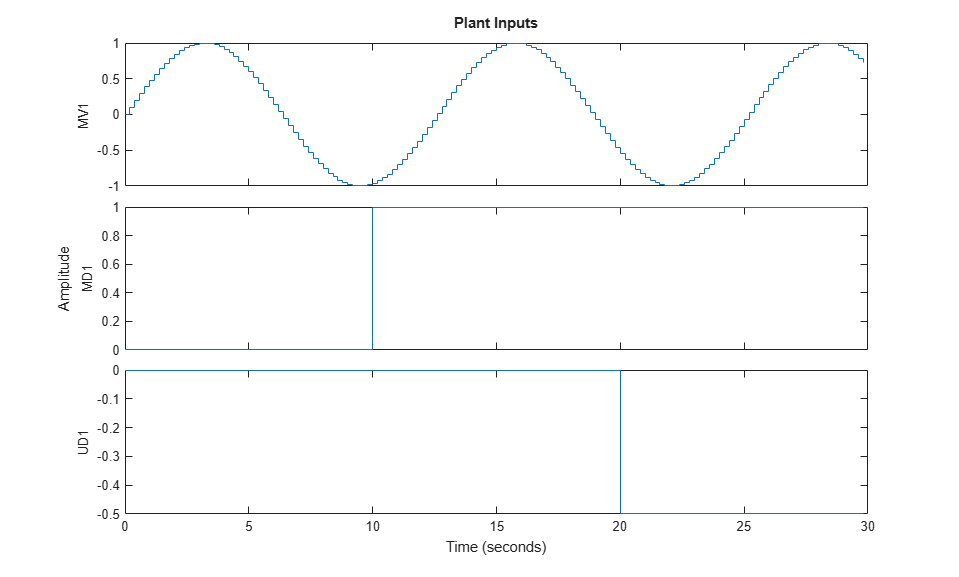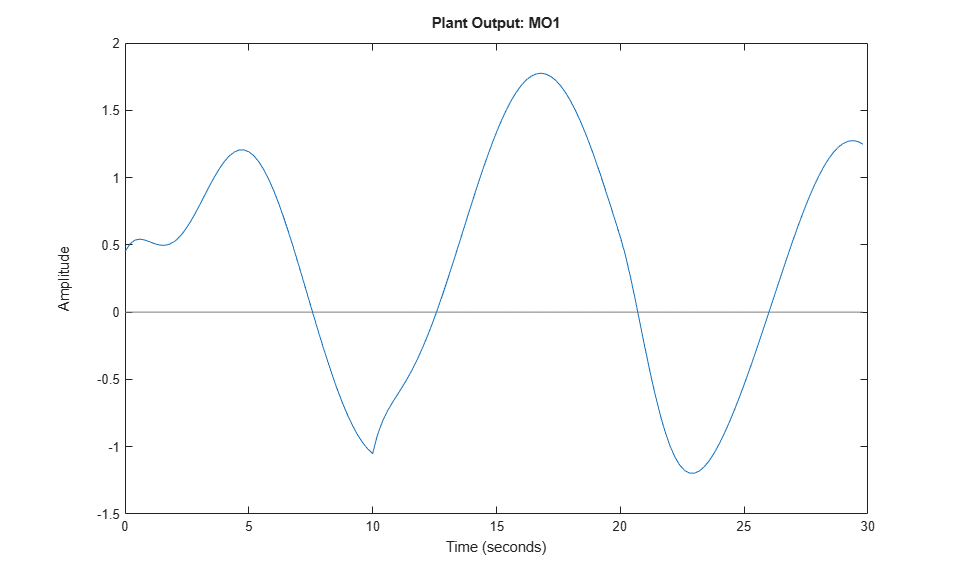MV constraint has been slightly violated while MO constraint has been violated more. Penalize more output constraint and rerun the simulation.

```mpcobj.OV.MaxECR = 0.001; % The closer to zero, the harder the constraint sim(mpcobj,Tf,r,v) ```
``` Assuming no disturbance added to measured output channel #1. -->The "Model.Noise" property of the "mpc" object is empty. Assuming white noise on each measured output channel. ```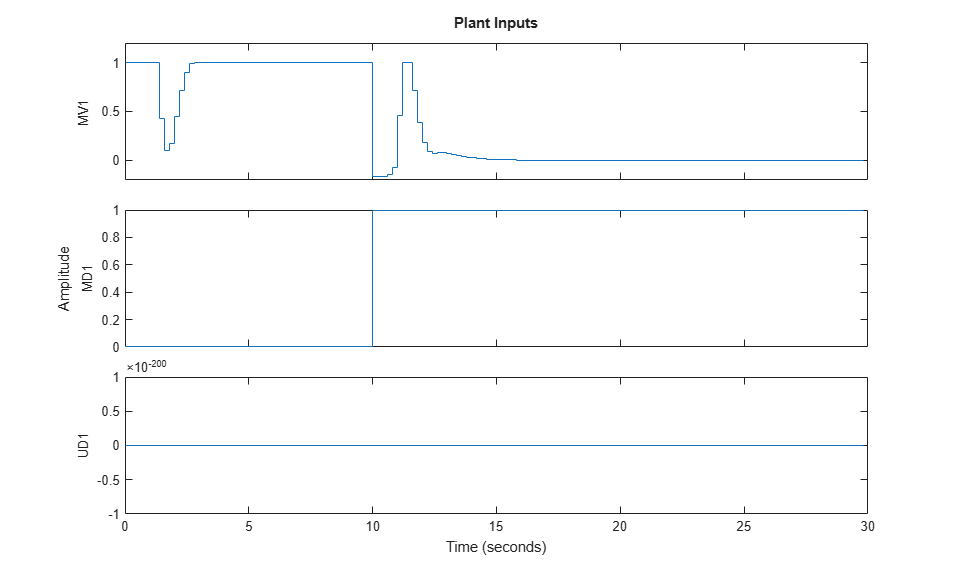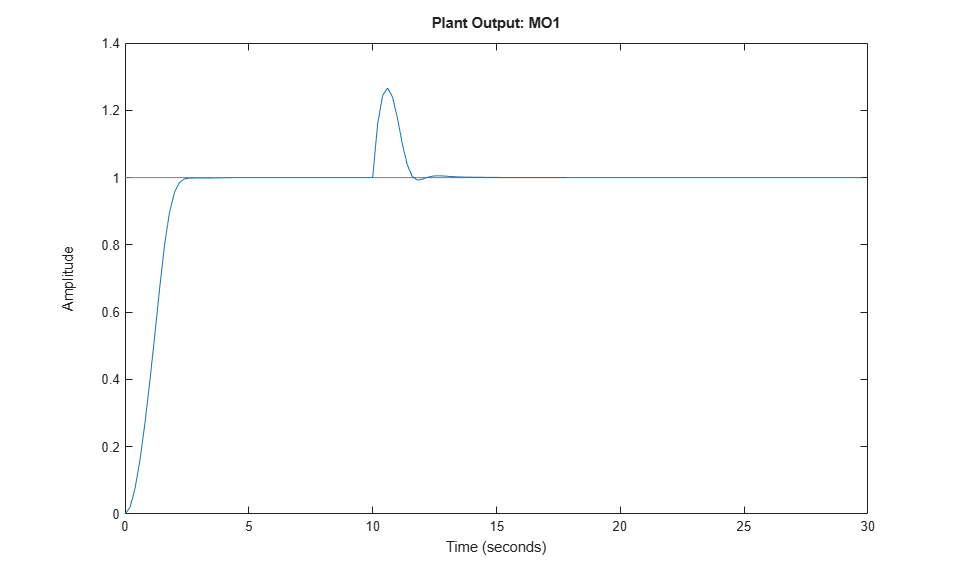Now MO constraint has been slightly violated while MV constraint has been violated more as expected.

### Change Kalman Gains Used in the Built-In State Estimator

Model Predictive Control Toolbox™ software provides a default Kalman filter to estimate the state of plant, disturbance, and noise models. You can change the Kalman gains.

First, retrieve the default Kalman gains and state-space matrices.

```[L,M,A1,Cm1] = getEstimator(mpcobj); ```

The default observer poles are:

```e = eig(A1-A1*M*Cm1) ```
```e = 0.5708 + 0.4144i 0.5708 - 0.4144i 0.4967 + 0.0000i 0.9334 + 0.1831i 0.9334 - 0.1831i 0.8189 + 0.0000i ```

Design a new state estimator by pole-placement.

```poles = [.8 .75 .7 .85 .6 .81]; L = place(A1',Cm1',poles)'; M = A1\L; setEstimator(mpcobj,L,M); ```

### Simulate Open-Loop Response

Test the behavior of plant in open-loop using the `sim` command. Set the `OpenLoop` flag to `on`, and provide the sequence of manipulated variables that excite the system.

```SimOptions.OpenLoop = 'on'; SimOptions.MVSignal = sin((0:Tf-1)'/10); ```

As the reference signal will be ignored, we can avoid specifying it.

```sim(mpcobj,Tf,[],v,SimOptions) ```
``` Assuming no disturbance added to measured output channel #1. -->The "Model.Noise" property of the "mpc" object is empty. Assuming white noise on each measured output channel. -->Converting model to discrete time. ```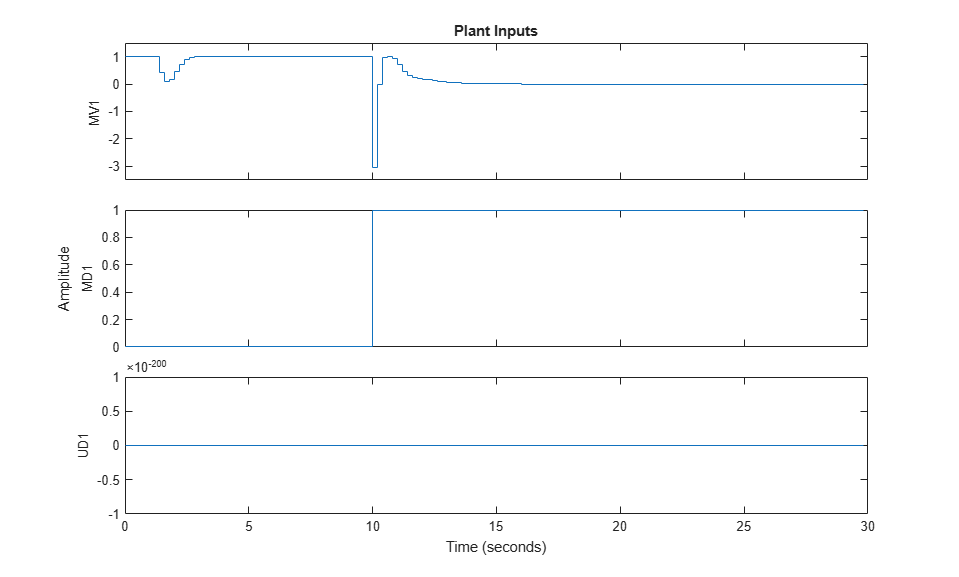To examine whether the MPC controller will be able to reject constant output disturbances and track constant setpoint with zero offsets in steady-state, compute the DC gain from output disturbances to controlled outputs using the `cloffset` command.

```DC = cloffset(mpcobj); fprintf('DC gain from output disturbance to output = %5.8f (=%g) \n',DC,DC); ```
```DC gain from output disturbance to output = 0.00000000 (=3.10862e-15) ```

A zero gain means that the output will track the desired setpoint.

### Simulate MPC with `mpcmove`

First, obtain the discrete-time state-space matrices of the plant.

```[A,B,C,D] = ssdata(model); Tstop = 5; % Simulation time x = [0 0 0 0 0]'; % Initial state of the plant xmpc = mpcstate(mpcobj); % Initial state of the MPC controller r = 1; % Output reference signal ```

Store the closed-loop MPC trajectories in arrays `YY`, `UU`, and `XX`.

```YY=[]; UU=[]; XX=[]; ```

Run the simulation loop

```for t=0:round(Tstop/Ts)-1 % Store states XX = [XX,x]; %#ok<*AGROW> % Define measured disturbance signal v = 0; if t*Ts>=10 v = 1; end % Define unmeasured disturbance signal d = 0; if t*Ts>=20 d = -0.5; end % Plant equations: output update (no feedthrough from MV to Y) y = C*x + D(:,2)*v + D(:,3)*d; YY = [YY,y]; % Compute MPC action u = mpcmove(mpcobj,xmpc,y,r,v); % Plant equations: state update x = A*x + B(:,1)*u + B(:,2)*v + B(:,3)*d; UU = [UU,u]; end ```

Plot the results.

```figure subplot(2,1,1) plot(0:Ts:Tstop-Ts,YY) grid title('Output') subplot(2,1,2) plot(0:Ts:Tstop-Ts,UU) grid title('Input') ```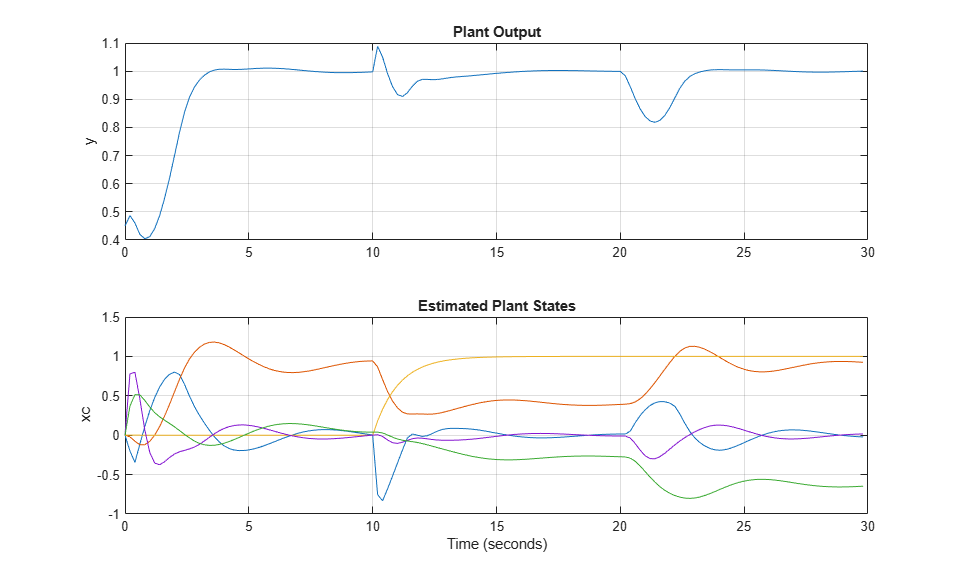If at any time during the simulation you want to check the optimal predicted trajectories, it can be returned by `mpcmove` as well. Assume you want to start from the current state and have a set-point change to 0.5, and assume the measured disturbance is gone.

```r = 0.5; v = 0; [~,Info] = mpcmove(mpcobj,xmpc,y,r,v); ```

We now extract the optimal predicted trajectories.

```topt = Info.Topt; yopt = Info.Yopt; uopt = Info.Uopt; figure subplot(2,1,1) stairs(topt,yopt) title('Optimal sequence of predicted outputs') grid subplot(2,1,2) stairs(topt,uopt) title('Optimal sequence of manipulated variables') grid ```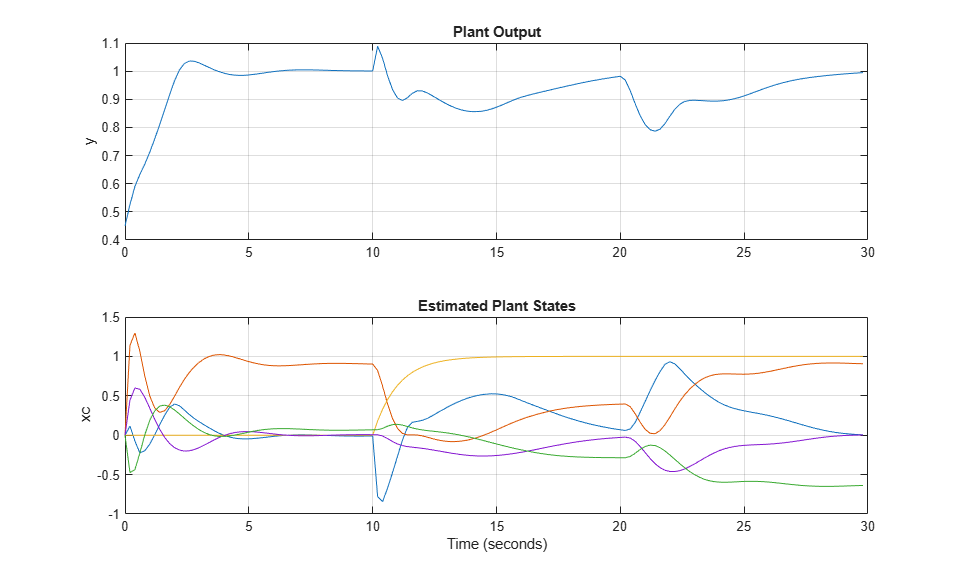### Linear Representation of MPC Controller

When the constraints are not active, MPC controller behaves like a linear controller. You can get the state-space form of the MPC controller.

```LTI = ss(mpcobj,'rv'); ```

Get state-space matrices of linearized controller.

```[AL,BL,CL,DL] = ssdata(LTI); ```

Simulate linear MPC closed-loop system and compare the linearized MPC controller with the original MPC controller with constraints turned off.

```mpcobj.MV = []; % No input constraints mpcobj.OV = []; % No output constraints Tstop = 5; %Simulation time xL = zeros(size(BL,1),1); % Initial state of linearized MPC controller x = [0 0 0 0 0]'; % Initial state of plant y = 0; % Initial measured output r = 1; % Output reference set-point u = 0; % Previous input command YY = []; XX = []; xmpc = mpcstate(mpcobj); for t = 0:round(Tstop/Ts)-1 YY = [YY,y]; XX = [XX,x]; v = 0; if t*Ts>=10 v = 1; end d = 0; if t*Ts>=20 d = -0.5; end uold = u; % Compute the linear MPC control action u = CL*xL+DL*[y;r;v]; % Compare the input move with the one provided by MPCMOVE uMPC = mpcmove(mpcobj,xmpc,y,r,v); dispStr(t+1) = {sprintf('t=%5.2f, u=%7.4f (provided by LTI), u=%7.4f (provided by MPCOBJ)',t*Ts,u,uMPC)}; %#ok<*SAGROW> % Update plant equations x = A*x + B(:,1)*u + B(:,2)*v + B(:,3)*d; % Update controller equations xL = AL*xL + BL*[y;r;v]; % Update output equations y = C*x + D(:,1)*u + D(:,2)*v + D(:,3)*d; end ```
``` Assuming no disturbance added to measured output channel #1. -->The "Model.Noise" property of the "mpc" object is empty. Assuming white noise on each measured output channel. ```

Display the results.

```for t=0:round(Tstop/Ts)-1 disp(dispStr{t+1}); end ```
```t= 0.00, u= 5.2478 (provided by LTI), u= 5.2478 (provided by MPCOBJ) t= 0.20, u= 3.0134 (provided by LTI), u= 3.0134 (provided by MPCOBJ) t= 0.40, u= 0.2281 (provided by LTI), u= 0.2281 (provided by MPCOBJ) t= 0.60, u=-0.9952 (provided by LTI), u=-0.9952 (provided by MPCOBJ) t= 0.80, u=-0.8749 (provided by LTI), u=-0.8749 (provided by MPCOBJ) t= 1.00, u=-0.2022 (provided by LTI), u=-0.2022 (provided by MPCOBJ) t= 1.20, u= 0.4459 (provided by LTI), u= 0.4459 (provided by MPCOBJ) t= 1.40, u= 0.8489 (provided by LTI), u= 0.8489 (provided by MPCOBJ) t= 1.60, u= 1.0192 (provided by LTI), u= 1.0192 (provided by MPCOBJ) t= 1.80, u= 1.0511 (provided by LTI), u= 1.0511 (provided by MPCOBJ) t= 2.00, u= 1.0304 (provided by LTI), u= 1.0304 (provided by MPCOBJ) t= 2.20, u= 1.0053 (provided by LTI), u= 1.0053 (provided by MPCOBJ) t= 2.40, u= 0.9920 (provided by LTI), u= 0.9920 (provided by MPCOBJ) t= 2.60, u= 0.9896 (provided by LTI), u= 0.9896 (provided by MPCOBJ) t= 2.80, u= 0.9925 (provided by LTI), u= 0.9925 (provided by MPCOBJ) t= 3.00, u= 0.9964 (provided by LTI), u= 0.9964 (provided by MPCOBJ) t= 3.20, u= 0.9990 (provided by LTI), u= 0.9990 (provided by MPCOBJ) t= 3.40, u= 1.0002 (provided by LTI), u= 1.0002 (provided by MPCOBJ) t= 3.60, u= 1.0004 (provided by LTI), u= 1.0004 (provided by MPCOBJ) t= 3.80, u= 1.0003 (provided by LTI), u= 1.0003 (provided by MPCOBJ) t= 4.00, u= 1.0001 (provided by LTI), u= 1.0001 (provided by MPCOBJ) t= 4.20, u= 1.0000 (provided by LTI), u= 1.0000 (provided by MPCOBJ) t= 4.40, u= 0.9999 (provided by LTI), u= 0.9999 (provided by MPCOBJ) t= 4.60, u= 1.0000 (provided by LTI), u= 1.0000 (provided by MPCOBJ) t= 4.80, u= 1.0000 (provided by LTI), u= 1.0000 (provided by MPCOBJ) ```

Plot the results.

```figure plot(0:Ts:Tstop-Ts,YY) grid ```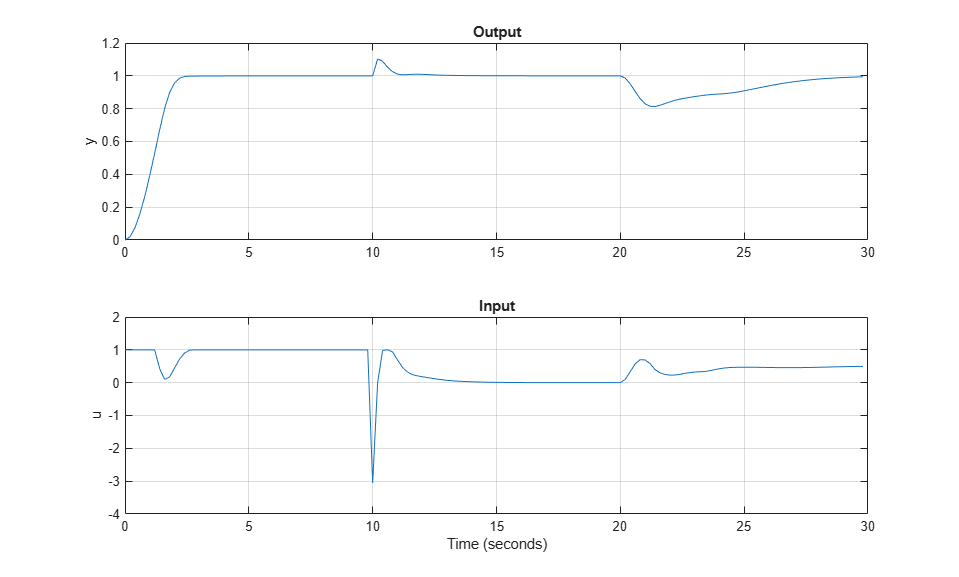Running a closed-loop where all constraints are turned off is easy using `sim`. We just specify an option in the SimOptions structure:

```SimOptions = mpcsimopt(mpcobj); SimOptions.Constr = 'off'; % Remove all MPC constraints SimOptions.Unmeas = d; % unmeasured input disturbance sim(mpcobj,Tf,r,v,SimOptions); ```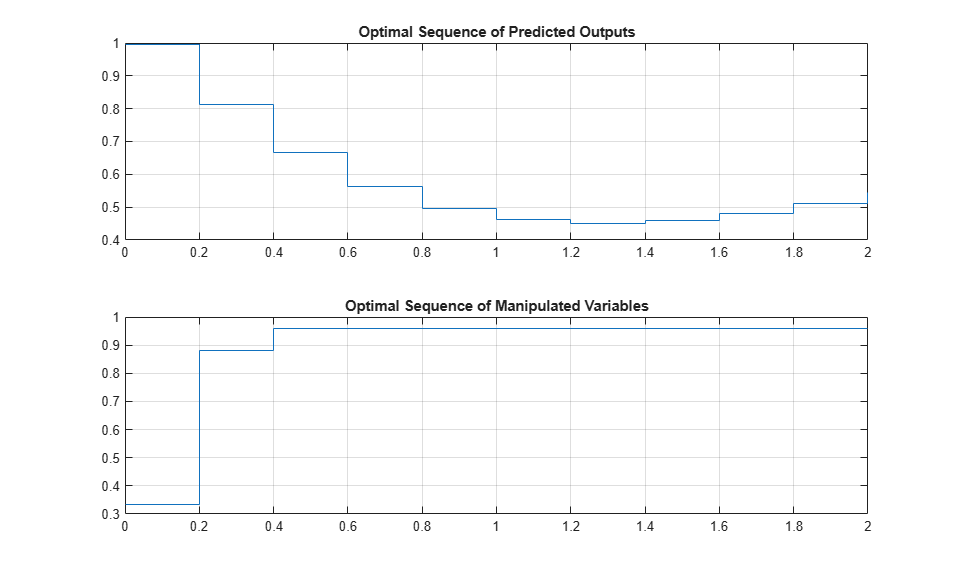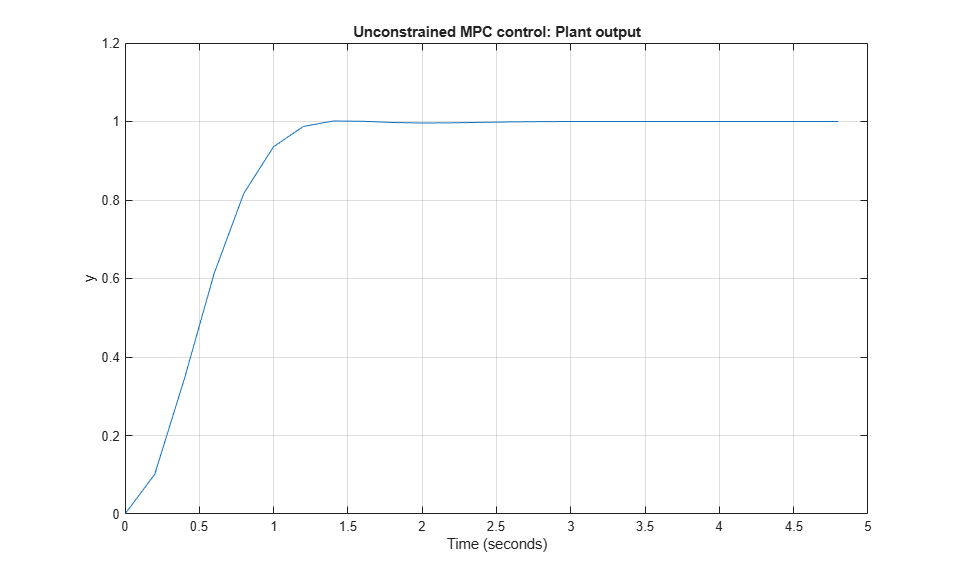To run this example, Simulink® is required.

```if ~mpcchecktoolboxinstalled('simulink') disp('Simulink(R) is required to run this part of the example.') return end ```

Recreate the MPC controller.

```mpcobj = mpc(model,Ts,10,3); mpcobj.MV = struct('Min',0,'Max',1,'RateMin',-10,'RateMax',10); mpcobj.Model.Disturbance = tf(sqrt(1000),[1 0]); ```
```-->The "Weights.ManipulatedVariables" property of "mpc" object is empty. Assuming default 0.00000. -->The "Weights.ManipulatedVariablesRate" property of "mpc" object is empty. Assuming default 0.10000. -->The "Weights.OutputVariables" property of "mpc" object is empty. Assuming default 1.00000. ```

The continuous-time plant to be controlled has the following state-space realization:

```[A,B,C,D] = ssdata(sys); ```

```mdl1 = 'mpc_miso'; open_system(mdl1) sim(mdl1) ```
``` Assuming no disturbance added to measured output channel #1. -->The "Model.Noise" property of the "mpc" object is empty. Assuming white noise on each measured output channel. ```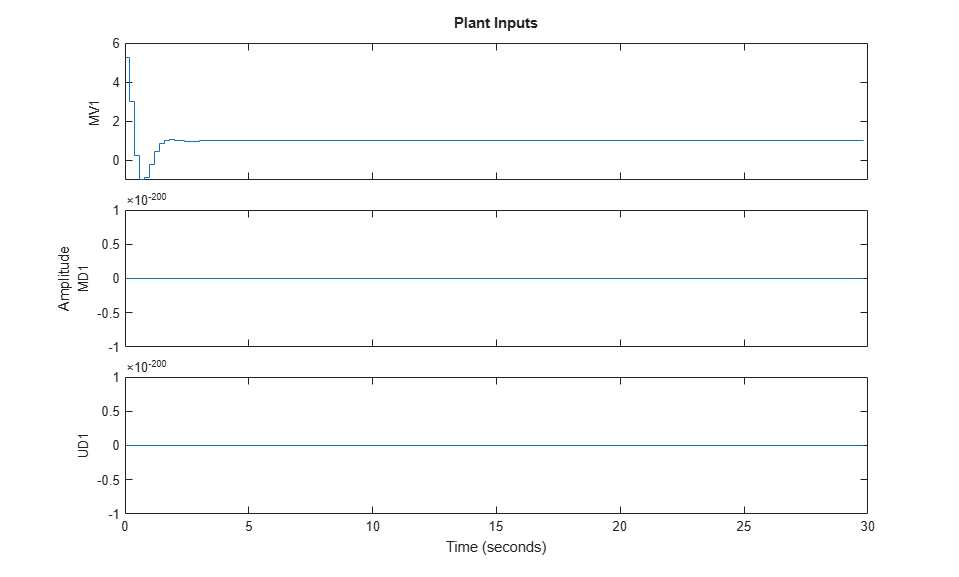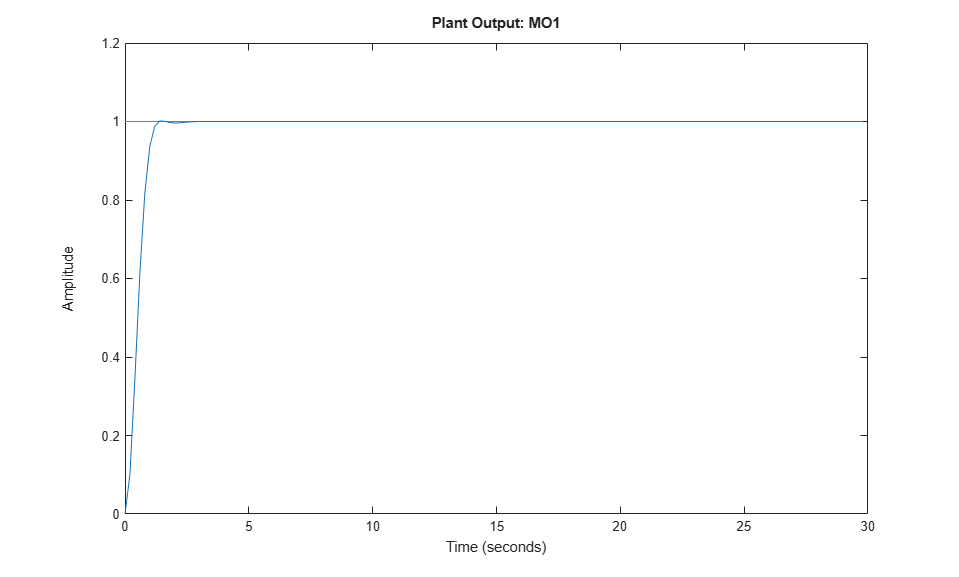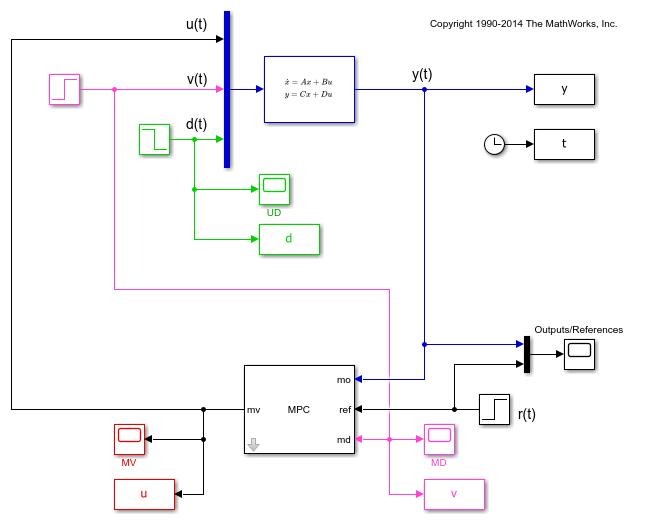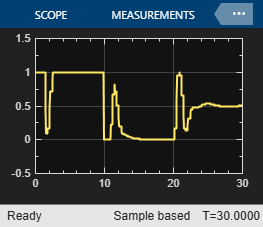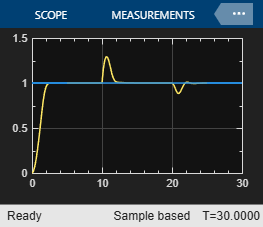Next, run a simulation with sinusoidal output noise. Assume output measurements are affected by a sinusoidal measurement noise of frequency 0.1 Hz. You want to inform the MPC object about this so that state estimates can be improved.

```omega = 2*pi/10; mpcobj.Model.Noise = 0.5*tf(omega^2,[1 0 omega^2]); ```

Revise the MPC design and specify a white Gaussian noise unmeasured disturbance with zero mean and variance `0.1`.

```mpcobj.Model.Disturbance = tf(0.1); mpcobj.weights = struct('MV',0,'MVRate',0.1,'OV',0.005); mpcobj.predictionhorizon = 40; mpcobj.controlhorizon = 3; ```

Run the simulation.

```Tstop = 150; mdl2 = 'mpc_misonoise'; open_system(mdl2) sim(mdl2,Tstop) ```
```-->Assuming output disturbance added to measured output channel #1 is integrated white noise. -->A feedthrough channel in NoiseModel was inserted to prevent problems with estimator design. ```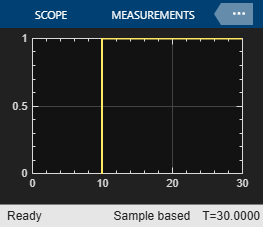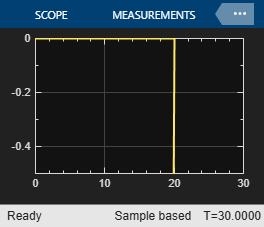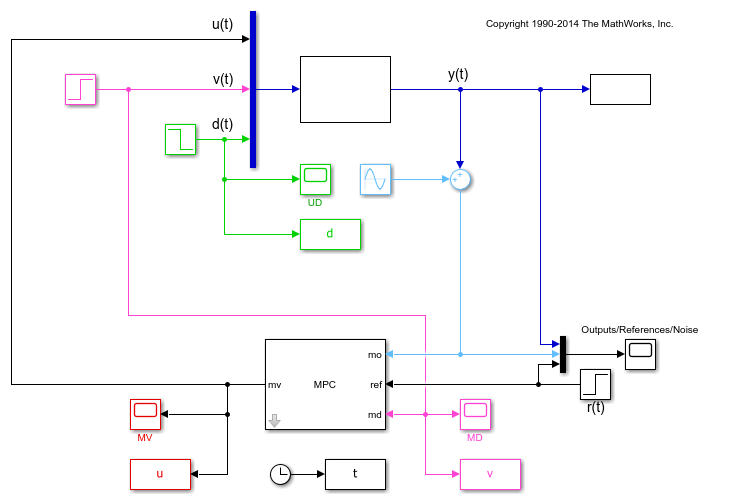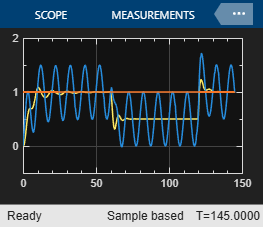```bdclose(mdl1) bdclose(mdl2) ```